# Linear Function Formula

• Last Updated : 16 Feb, 2022

A linear function is a process that permits the description of the straight line on the coordinate plane. For example, y = 2x – 1 designates the straight line on the coordinate plane, and therefore it conveys a linear process. As y can be substituted by f(x), this process can be registered as f(x) = 2x – 1.

It is one of the state f(x) = mx + b , here ‘m’ and ‘b’ denotes the real numbers. It’s examining like the slope-intercept shape of a bar which is provided by y = mx + b because the linear process denotes a bar i.e. it’s a graph line. Here,

‘m’ is the pitch of the string

‘b’ is the y-intercept of the bar

‘x’ is the separate variable

‘y’ (or f(x)) is the conditional variable

A linear procedure is also known as an algebraic procedure.

Example of linear functions

f(x) = 2x + 1

f(x) = -3x – 2

f(x) = 5

The linear function formula is used to represent the objective function of the linear programming problems, which helps to maximize profits.

### Linear function Formula

The data is provided as a graph about a process, it is linear if the graph is a bar. If the data is provided in the state of algebraic structure about the function, then it is linear if it is of the form f(x) = mx+b. But to see whether the shared data in a table form describe a linear equation or not we have to,

• Calculate the distinctions in y-values

• Calculate the distinctions in the x-axis

• Check whether the balance of the dissimilarity in y-values to the dissimilarity in x-values is always steady.

Graphing of a linear function

As we comprehend to plot a line on a graph we require any two points on it. If we discover two points we only have to devise them on the graph merge them by a line and spread from both flanks. The graph of a linear function f(x) = mx + b is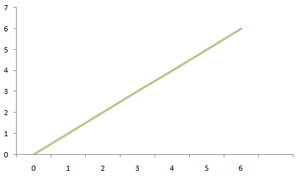increasing line when m >0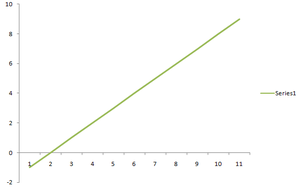decreasing line when m < 0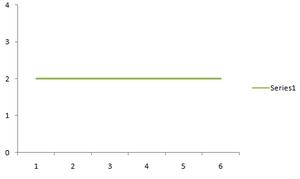horizontal line when m = 0

Graphing a linear function by finding two points

To discover two pinpoints on a linear function (line) f(x)=mx+b, consider some unexpected values for ‘x’ and have to replace these values to find the connected values of y.

This method is presented by an instance where we are proceeding to graph the function f(x) = 2x + 4.

Step1: Firstly find two points on the line by carrying some unexpected values

assume that x = 0 and x = 1

Step2: Now replace each of both the values in position to get the related y-values

Here is the table of a linear procedure y = 2x + 4

So, the two points on the line are (0, 4) and (1, 6).

Step3: Now plan the points on the graph merge them by the line and expand the line from both sides.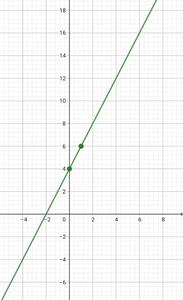Graphing of linear function using slope and y-intercept

To graph a linear function , f(x)=mx+b, use its slope ‘m’ and its y-intercept ‘b’ .This procedure is explained again by graphing the same linear function f(x) = 2x + 4. Its slope is, m = 2 and its y-intercept is (0, b) = (0, 4).

Step 1: Firstly Plot the y-intercept (0, b).

Here, plot the point (0, 4).

Step2: Now document the slope as the fraction uprise/run and recognize the “uprise” and the “run”.

Here, the slope = 2 = 2/1 = uprise/run.

So uprise = 2 and run = 1.

Step3: Now uprise the y-intercept vertically by “rise” and then run horizontally by “run”. This will result in making a new point.

(Note that if “rise” is favorable, go up and if “rise” is unfavorable, go down. Also, if “run” is favorable”, go right, and if “run” is unfavorable, go left.)

Here, go up by 2 units from the y-intercept and thereby go right by 1 unit.

Step4: Now merge the points from step1 and step2 by line and expand that line on both sides.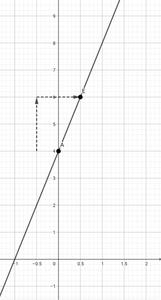Domain and range of linear function

The domain of the linear function is the collection of all real numbers, and actually, the scope of a linear function is also the collection of all-natural numerals figures show f(x) = 2x + 3 and g(x) = 4 −x devised on the same axes.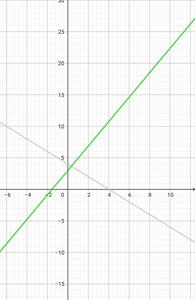Note: both the roles taken on the absolute value for all the values of x, mean that the part of each function is the collection of all-natural numerals (R). Examine along the x-axis to ensure. For every value of x, we have a moment on the graph.

Even, the result for each of the procedures varies from negative to positive infinity, which represents the scope of either function is also R. That can be verified by examining along the y-axis, which apparently delivers that there is a moment on each graph for every y-value. Thus, when the slope m ≠ 0,

•The parts of a linear function = R

•The scope of a linear function = R

Note:

(i) When the slope, m = 0, then the linear function f(x) = b is a horizontal line and in this case, the fields = R and the scope = {b}.

(ii) The fields and scope of a linear function in R as extended as the issue has not cited any exact field or scope.

The inverse of a linear function

Inverse of the linear function f(x) = ax + b is shown by a function f-1(x) such that f(f-1(x)) = f-1(f(x)) = x.

The method of seeing the inverse of a linear function is presented through an instance where we are heading to find the inverse of a function f(x) = 2x + 4.

Step1: Write y instead of f(x)

So, equation y = 2x + 4

Step2: Now interchange the variable x and y

Then we have x = 2y + 4

Step3: Solve the above equation to get y

x – 4 = 2y

y = (x – 4)/2

Step4: Replace y by f-1(x) and it is the inverse function of f(x).

f-1(x) = (x – 4)/2

Note:- that the f(x) and f-1(x)are always symmetric with respect to the line y=x. Let us plot the linear function f(x) = 2x + 5 and its inverse f-1(x) = (x – 4)/2 and see whether they are symmetric about y = x or not . Also when (x, y) lies on f(x) , then (y, x) lies on f-1(x). For example, in the following graph (-1, 2) lies on f(x) where as (2, -1) lies on f-1(x).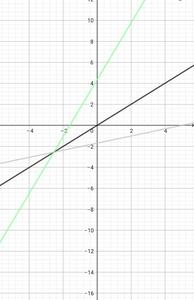Piecewise linear function

Periodically the linear function may not be described uniformly via its habitat. It may be described in two better routes as its habitat separates in two or more methods. In these changes, it is understood as a piecewise linear function. Let’s take a sample.

### Sample Problems

Question 1: Find the linear function that has two points (-2, 17) and (1, 26) on it.

Solution:

The given points are (x1, y1)= (-2, 17) and (x2, y2) = (1, 26)

Step1: Firstly find the slope of the function using the slope formula:

m = (y₂ – y₁) / (x₂ – x₁) = (26 – 17) / (1 – (-2)) = 9/3 = 3.

Step2: Now find the equation of linear function using the point slope form

y – y₁ = m (x – x₁)

y – 17 = 3 (x – (-2))

y – 17 = 4 (x + 2)

y – 17 = 4x + 8

y = 4x + 25

So, the equation of linear function is, f(x) = 4x + 25

Question 2: Decide whether the subsequent data from the subsequent table describes a linear function.

Solution:

We have to compute the differences in the x-values, differences in the y-values, and the ratio by (difference in y/difference in x) and have to see whether this ratio is constant or not.

Since all the numbers in the last column are equal to constant, the given table represents the linear function.

Question 3: Plot graph y = 3x + 2 is a linear equation

Solution: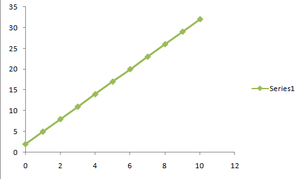When x increases, y increases thrice as fast, so we need 3x.

When x is 0, y is already 2. So +2 is also needed.

And so: y = 3x + 2

Here are some example values:

Question 4: Plot the graph of the following equation 3x + 2y − 4 = 0

Solution:

It is in the form Ax + By + C = 0 where:

A = 3

B = 2

C = −4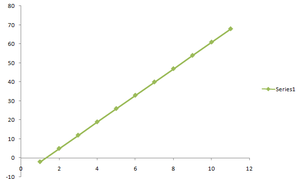Question 5: Plot the graph of the following piecewise linear function.

• f(x) = x + 4,  x∈ [-3, -2, -1, 0, 1, 2]
• f(x) = 1x – 2, x∈ [-3, -2, -1, 0, 1, 2]

Solution:

The piece wise linear function indicates both the parts of its domain. Let us find the endpoint in each of the case.

• When f(x) = x + 4
• When  f(x) =1x-2

The corresponding graph is shown below.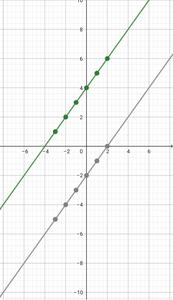My Personal Notes arrow_drop_up
Recommended Articles
Page :